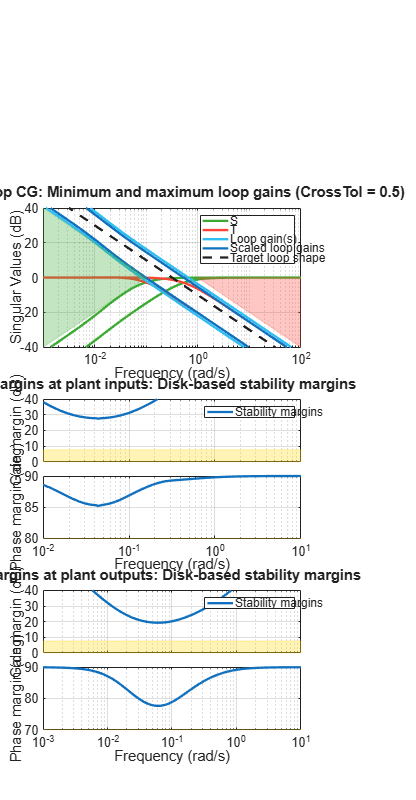# loopview

Graphically analyze MIMO feedback loops

## Syntax

``loopview(G,C)``
``loopview(G,C,info)``

## Description

example

````loopview(G,C)` plots characteristics of the following positive-feedback, multi-input, multi-output (MIMO) feedback loop with plant `G` and controller `C`.Use `loopview` to analyze the performance of a tuned control system you obtain using `looptune`. NoteIf you are tuning a Simulink® model with `looptune` through an `slTuner` interface, analyze the performance of your control system using `loopview` (Simulink Control Design) for `slTuner` (requires Simulink Control Design™). `loopview` plots the singular values of: Open-loop frequency responses `G*C` and `C*G`Sensitivity function `S = inv(1-G*C)` and complementary sensitivity ```T = 1-S```Maximum (target), actual (tuned), and normalized MIMO stability margins. `loopview` plots the multi-loop disk margin (see Stability Analysis Using Disk Margins (Robust Control Toolbox)). Use this plot to verify that the stability margins of the tuned system do not significantly exceed the target value.For more information about singular values, see `sigma`.```
````loopview(G,C,info)` uses the `info` structure returned by `looptune`. This syntax also plots the target and tuned values of tuning constraints imposed on the system. Additional plots include:Singular values of the maximum allowed `S` and `T`. The curve marked `S/T Max` shows the maximum allowed `S` on the low-frequency side of the plot, and the maximum allowed `T` on the high-frequency side. These curves are the constraints that `looptune` imposes on `S` and `T` to enforce the target crossover range `wc`.Target and tuned values of constraints imposed by any tuning goal requirements you used with `looptune`.Use `loopview` with the `info` structure to assist in troubleshooting when tuning fails to meet all requirements.```

## Examples

collapse all

Tune a control system with `looptune` and use `loopview` to examine the performance of the tuned controller.

Create and tune control system.

```s = tf('s'); G = 1/(75*s+1)*[87.8 -86.4; 108.2 -109.6]; G.InputName = {'qL','qV'}; G.OutputName = 'y'; D = tunableGain('Decoupler',eye(2)); PI_L = tunablePID('PI_L','pi'); PI_L.OutputName = 'qL'; PI_V = tunablePID('PI_V','pi'); PI_V.OutputName = 'qV'; sum = sumblk('e = r - y',2); C0 = (blkdiag(PI_L,PI_V)*D)*sum; wc = [0.1,1]; options = looptuneOptions('RandomStart',5); [G,C,gam,info] = looptune(-G,C0,wc,options);```
```Final: Peak gain = 0.956, Iterations = 28 Achieved target gain value TargetGain=1. ```

Examine the controller performance.

```figure('Position',[100,100,520,1000]) loopview(G,C,info)```The first plot shows that the open-loop gain crossovers fall close to the specified interval [0.1,1]. This plot also includes the tuned values of the sensitivity function `S = inv(1-G*C)` and complementary sensitivity `T = 1-S`. These curves reflect the constraints that `looptune` imposes on `S` and `T` to enforce the target crossover range `wc`.

The second and third plots show that the MIMO stability margins of the tuned system fall well within the target range.

## Input Arguments

collapse all

Plant in a control system, specified as a Numeric LTI model or tunable `genss` model.

The plant is the portion of your control system whose outputs are sensor signals (measurements) and whose inputs are actuator signals (controls).

You can obtain `G` as an output argument from the `looptune` function when you tune your control system.

Controller in a control system, specified as a Generalized LTI model model.

The controller is the portion of your control system that receives sensor signals (measurements) as inputs and produces actuator signals (controls) as outputs.

You can obtain `C` as an output argument from `looptune` when you tune your control system.

`info` structure returned by `looptune` during control system tuning.

## Alternatives

For analyzing Simulink models tuned with `looptune` through an `slTuner` (Simulink Control Design) interface, use `loopview` (Simulink Control Design) for `slTuner` (requires Simulink Control Design).